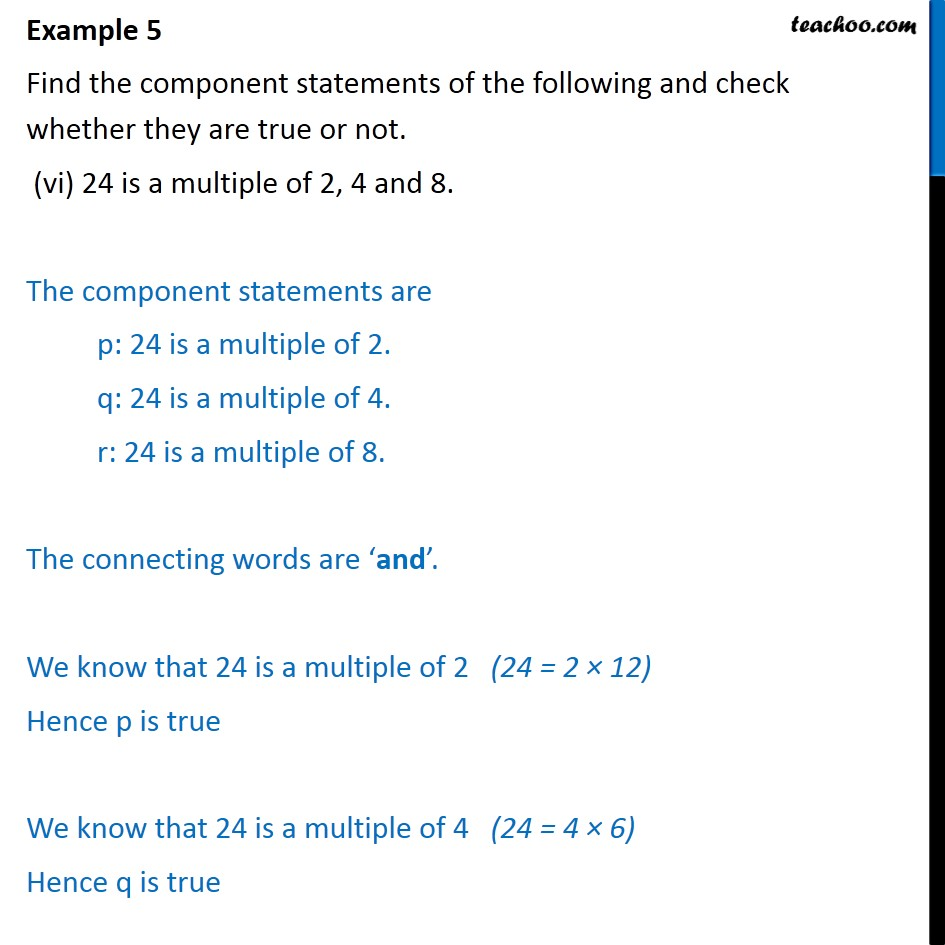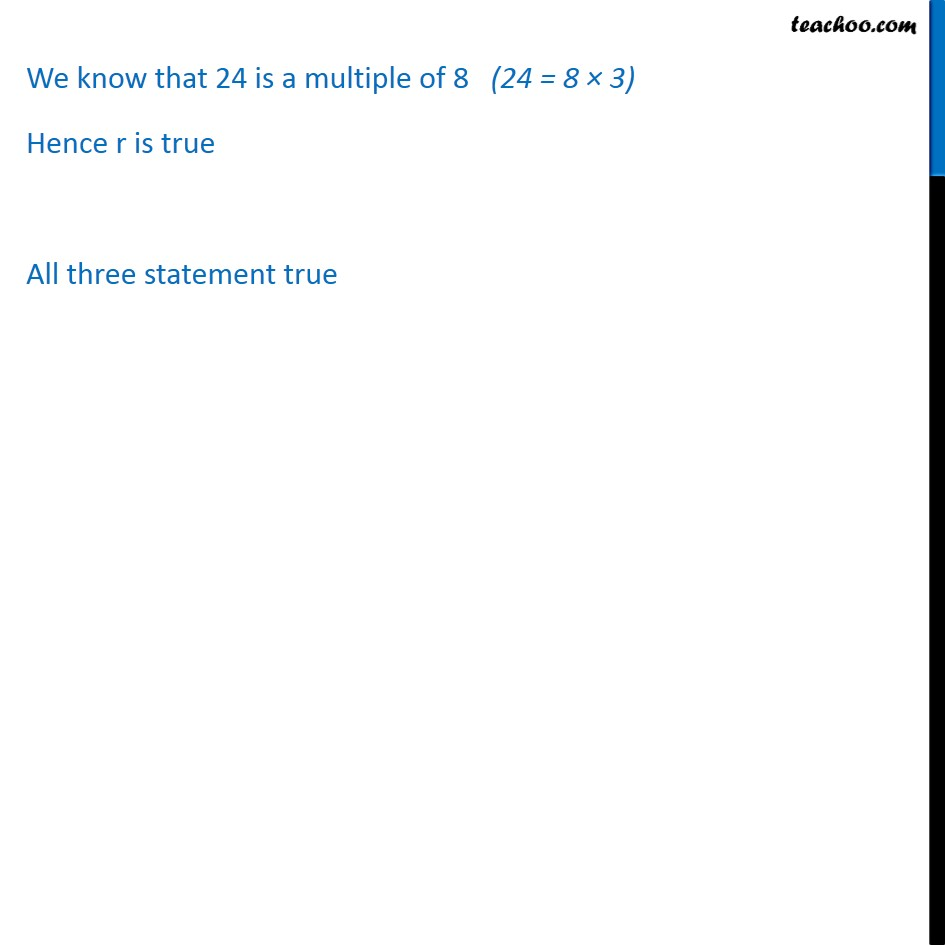Examples

Chapter 14 Class 11 Mathematical Reasoning
Serial order wiseIntroducing your new favourite teacher - Teachoo Black, at only ₹83 per month

### Transcript

Example 5 Find the component statements of the following and check whether they are true or not. (vi) 24 is a multiple of 2, 4 and 8. The component statements are p: 24 is a multiple of 2. q: 24 is a multiple of 4. r: 24 is a multiple of 8. The connecting words are and . We know that 24 is a multiple of 2 (24 = 2 12) Hence p is true We know that 24 is a multiple of 4 (24 = 4 6) Hence q is true We know that 24 is a multiple of 8 (24 = 8 3) Hence r is true All three statement true